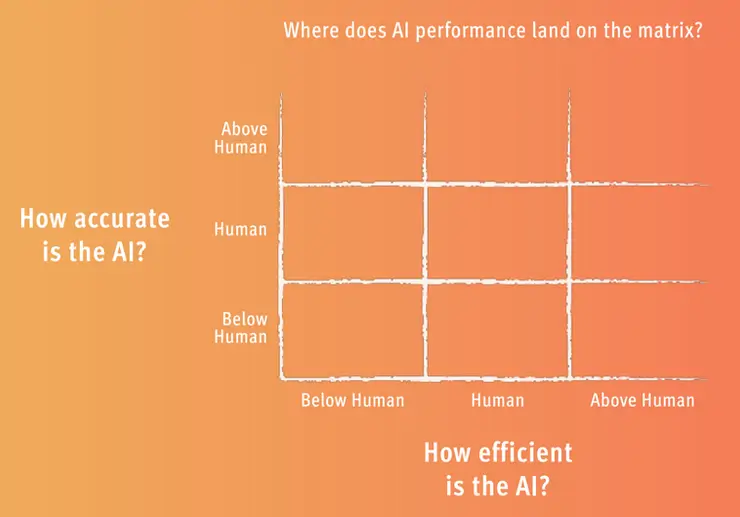If we use accuracy and efficiency as our key metrics to compare AI and human performance, we need to put them into a matrix. A simple way to do this is to create performance bands. If we take human performance as the benchmark, we can create adjacent bands for below-human performance, and above-human performance.

We can then use the matrix (see below) to plot where AI performance lands on this scale. The benefit of this matrix is that it can be applied to many different contexts, and the standards for below-human, human, and above-human are relative to each task.

Let’s look at a tangible example